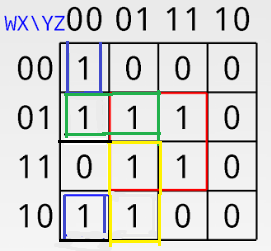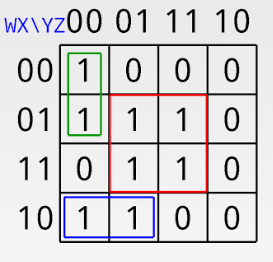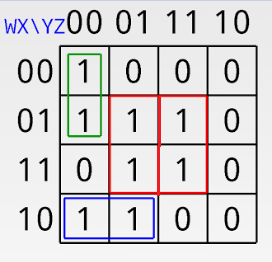# GATE | GATE-CS-2007 | Question 85

Let f(w, x, y, z) = ∑(0, 4, 5, 7, 8, 9, 13, 15). Which of the following expressions are NOT equivalent to f?
(A) x’y’z’ + w’xy’ + wy’z + xz
(B) w’y’z’ + wx’y’ + xz
(C) w’y’z’ + wx’y’ + xyz + xy’z
(D) x’y’z’ + wx’y’ + w’y

Explanation:Solving this k-map we get x’y’z’ + w’xy’ + wy’z + xz which is (A) partSolving this k-map we get w’y’z’ + wx’y’ + xz which is (B) part.Solving this k-map we get w’y’z’ + wx’y’ + xyz + xy’z which is ( C) part .

But we can’t get (D) part from any combination so Ans is (D) part.

Quiz of this Question